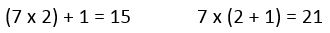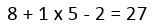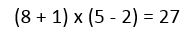# 11-Plus Exam Illustrations - Maths Quiz - Prime Numbers and Using Brackets in Sums (Questions)

## Prime Numbers

Prime numbers are those which can only be divided by themselves and one.

The sequence made by the first prime numbers is useful to learn but they are simply unconnected numbers which don't follow a special pattern.

One, as it is only divisible by one (which is itself), is not considered a prime number.

Two, as it is only divisible by one and itself, is a prime number and the only EVEN prime number.

Here are the first primes:

 2 3 5 7 11 13 17 19 23 29 31 37 41

As you can see, there is no pattern and this list would go on forever.

You cannot really work out whether a certain number will be a prime or not without going through a lot of trial and error. Prime numbers serve a purpose in doing some mathematical tasks but in general you will only be expecting to get your child to recognise them and know what they are. They may be asked to find a prime number within a certain range or knowing the answer is a prime number may exclude an alternative one from a sum. Another possibility is to find the 'next prime number after ...'

For example:

What is the nearest prime to 100?

The nearest numbers are 99 and 101. We can rule out 99 as it is in the three times table. 101 does not fit into any times tables so it is prime.

A good rule of thumb is to find the nearest square number and establish the square root. In this case it is 100 and the square root is ten. This means that we only need to search for possible factors up to 10. If 101 is not in any times tables up to 10, it certainly won't be in any higher ones. Remember that the factors work in pairs so if you thought 17 was a factor, it would have to be matched with a number below 10. If you've already ruled out all the numbers up to 10, this cannot be the case.

## Using Brackets in Sums

Brackets are very useful in sums. Regardless of whether you are applying the rules of BIDMAS (whether to carry out division before multiplication, etc) you should always carry out the sum in brackets before you do anything else.

For example, consider the difference between the following:The difference is that the part in brackets is being calculated first. In the first sum, (7 x 2) is calculated before the 1 is added on. In the second sum, (2 + 1) is calculated and then multiplied by 7.

We carry out the section in brackets before anything else; if there are two sets of brackets, or brackets within brackets, each one must be done separately before any other sections of the sum.

So, how will your child encounter these in a maths test? Probably something like this:

Make the following sum correct by adding brackets:If we read it in order and calculate each step at a time, the answer will be 43. If we use BIDMAS and follow the rules of which part to do first, (multiplication should come before the addition and subtraction), the answer will be 11.

So, how can brackets make the sum correct? We cannot rearrange the numbers nor change any of them. Brackets must go around pairs of numbers thus:It's simply a matter of trial and error; bracket off each section of the sum and see whether it has a connection to the answer. In this case, 8 + 1 = 9 and we know that 9 times 3 is 27, so that's a promising start. 5 - 2 = 3 so the sum now works.

Becoming familiar with how to carry out calculations using BIDMAS takes a lot of practice. For a detailed look at how BIDMAS (sometimes called BODMAS) works, have a read of our Using BIDMAS in calculations page.